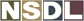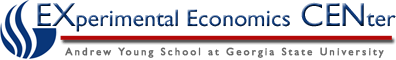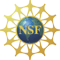Students / Subjects# Price Index

Since Inflation is broadly defined as an increase in the general price level, in order to accurately measure inflation we must first assess the general price level.  The general price level is measured by a price index. A price index is a weighted average of the prices of a selected basket of goods and services relative to their prices in some base-year.

To construct a price index we start by selecting a base year. Then we take a representative sample of goods and services and calculate their value in the base year and current prices. The ratio of the expenditures on the basket of goods at current prices to the expenditure at the base year prices is taken as the price index.

For example, suppose our basket of goods consists of only three items: shirts, pants and bread with the following prices and quantities in 2006 and 2007:

 Item Quantity Price in 2006 Price in 2007 Exp. in 2006 Exp. in 2007 Shirts 10 \$10 \$12 \$100 \$120 Pants 5 \$20 \$25 \$100 \$125 Bread 100 \$0.50 \$0.55 \$50 \$55 TOTAL \$250 \$300

We're now going to calculate the Market Basket values for 2006  and 2007.  Values that indicate Quantity will be in bold.

Market Basket for 2006 = (10* \$10) + (5* \$20) + (100* \$0.50) = \$100 + \$100 +\$50 = \$250

Market Basket for 2007 = (10* \$12) + (5* \$25) + (100* \$0.55) = \$120 + \$125 + \$55 = \$300

Notice that the same quantities were used for both calculations.  Undoubtedly the quantities of good would change year to year, however we want to hold these quantities constant so we can see the impact of the price changes.

To calculate the Price Index, take the price of the Market Basket of the year of interest and divide by the price of the Market Basket of the base year, then multiply by 100.  In this case we're interested in knowing the price index for 2007 and we plan to use 2006 as the base year.

Price Index for 2007= Market Basket for 2007 * 100 = 300  * 100 =  120